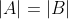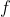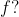# DM - Functions & Relations -Q4

+1 vote

Two sets X and Y are said to have the same cardinality if and only if there is a one-to-one correspondence(i.e. Bijection) from X to Y. When A and B have the same cardinality, we write |X|=|Y|.

Let A and B be two sets such that. There is a one to one functionfrom A to B.

Which of the following must be true forS1:is onto function

S2:has an inverse

(A). Only S1

(B). Only S2

(C). Both S1 and S2

(D). None of the aboveasked Jun 24
edited 3 days agoAbove function is one-one but not onto.

https://en.wikipedia.org/wiki/Cardinality#:~:text=Two%20sets%20A%20and%20B,%E2%89%88%20B%20or%20A%20~%20B

https://youtu.be/k7qtU5HvGtM

Watch this video start time 13:30 mint

D is correct one

NOTE that if A or B is a finite set then Option C would be the answer.answered 4 days ago by (112,390 points)
Sir but why we have considered here infinite sets ? We are given both contains same number of elements then why not go with finite sets?
Read Kenetha Rosen (Set Chapter, Cardinality Topic)

The sets A and B have the same cardinality if and only if there is a one-to-one correspondence from A to B. When A and B have the same cardinality, we write |A|=|B|.

For infinite sets the definition of cardinality provides a relative measure of the sizes of two sets,
rather than a measure of the size of one particular set.
i have a doubt, what if  There is a onto mapping from function f from A to B.

Which of the following must be true for f?

S1: f is one-one function

S2: f has an inverse

(A). Only S1

(B). Only S2

(C). Both S1 and S2

(D). None of the above
Is this FULL Question ?? Or would you like to put some more conditions ?

Because if it is FULL question in itself then answer is D. Function being ONTO doesn't mean that it is one-one Or have inverse.
yes this is the full question, why being onto does not imply one-one here.

suppose we have a mapping from natural numbers to whole numbers, since cardinalities are same and A->B is onto, so every element of B should have a pre-image, and also we cant leave out any elements in A because then it would not be a function.

Can you provide a counter example for this, A->B is onto but not one-one when
|A| = |B|
When |A| = |B| and assume A is finite set then every onto function is also one-one.
But assume A is set of all natural numbers(assuming 0 is Not a natural number) ,B is set of whole numbers. Now, consider this function f:B--> A,  f(n) = n, when n is non-zero and f(0)=1.  This is onto but not one-one. And note that |A| = |B| because both A,B are countable infinite.
ok got it, thanks